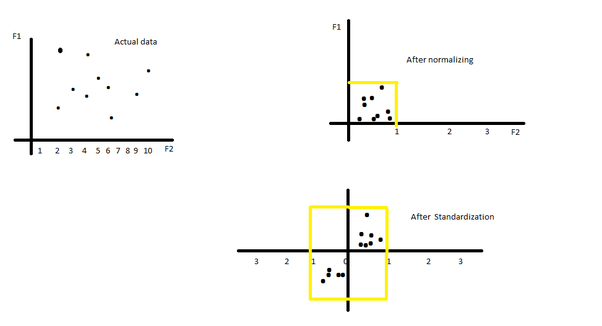/  Top Machine learning interview questions and answers   /  Explain in detail about Normalization and Standardization?## Explain in detail about Normalization and Standardization?

### Standardization

Standardization is the process of rescaling the features so that they’ll have the properties of a Gaussian distribution with

μ=0 and σ=1

where μ is the mean and σ is the standard deviation from the mean; standard scores (also called z scores) of the samples are calculated as follows:### Normalization

Normalization often also simply called Min-Max scaling shrinks the range of the data such that the range is fixed between 0 and 1. It works better for cases in which the standardization might not work so well. If the distribution is not Gaussian or the standard deviation is very small, the min-max scaler works better.